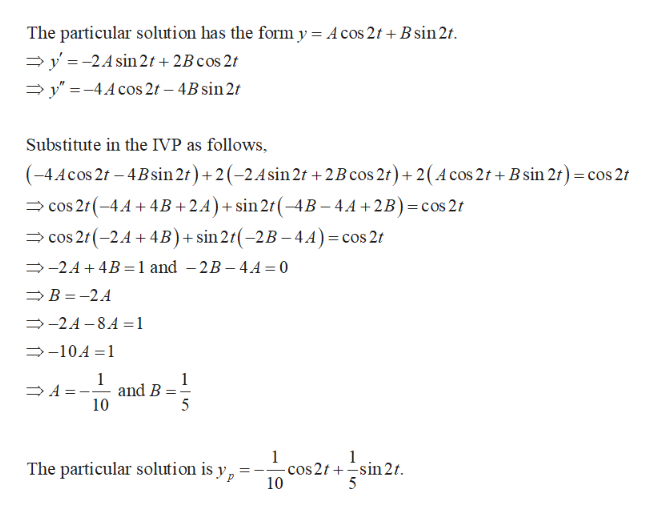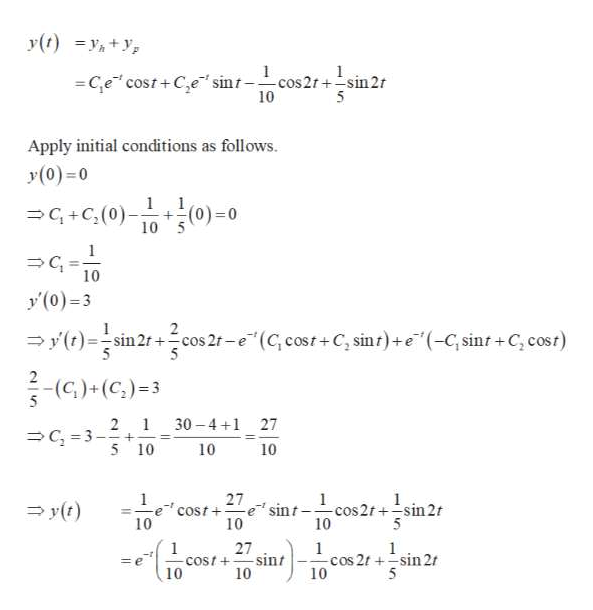# What is the transient solution of the following vibrating system:y'' + 2y' + 2y = cos 2t, y(0) = 0, y'(0) = 3

Question
7 views

What is the transient solution of the following vibrating system:

y'' + 2y' + 2y = cos 2t, y(0) = 0, y'(0) = 3

check_circle

Step 1

Find the complementary solution of the given initial value problem as follows.

Step 2

Find the particular solution of the given initial value problem as follows.help_outlineImage TranscriptioncloseThe particular solution has the form y = Acos 2f+ B sin 2t y'-24 sin 2 +2B cos 2f y-4Acos 2t -4Bsin 2/ Substitute in the IVP as follows, (-4Acos 21 -4Bsin 2r) + 2 (-2.4sin2t +2B cos 2t) +2( cos 2t + Bsin 2r) =cos 21 cos 2r(-4A4B +2.A)+sin 21(-4B - 4A +2 B) = cos 2t cos 2f (-2A 4B)+ sin21(-2B-4A) =cos 2t -2.A 4B=1 and -2B-4A = 0 B -2A -2A 8.A= 1 -104 1 1 and B 10 1 A = 1 cos 2t 10 1 sin 2 t. The particular solution is y, fullscreen
Step 3

Find the general solut...help_outlineImage Transcriptionclosey(t) 1 1 C,e cost+Ce sint-cos2t +sin2r 10 5 Apply initial conditions as follows y(0)=0 1 +(0)-0 10 5 1 G+C(0) C 10 y'(0)-3 y(t)sin 2cos 2f-e(C, cost+C, sint)+e(-C,sint+C, cost) 2 - (C)+(C,)3 5 2 1 C 3-+ 5 10 30 4 1 27 10 10 1 27 1 cos2t+-sin 2t 10 1 y0) e' sint cost + 10 10 5 27 sint COst+ 10 1 1 1 cos 2t sin 2 10 = e 10 fullscreen

### Want to see the full answer?

See Solution

#### Want to see this answer and more?

Solutions are written by subject experts who are available 24/7. Questions are typically answered within 1 hour.*

See Solution
*Response times may vary by subject and question.
Tagged in

### Math